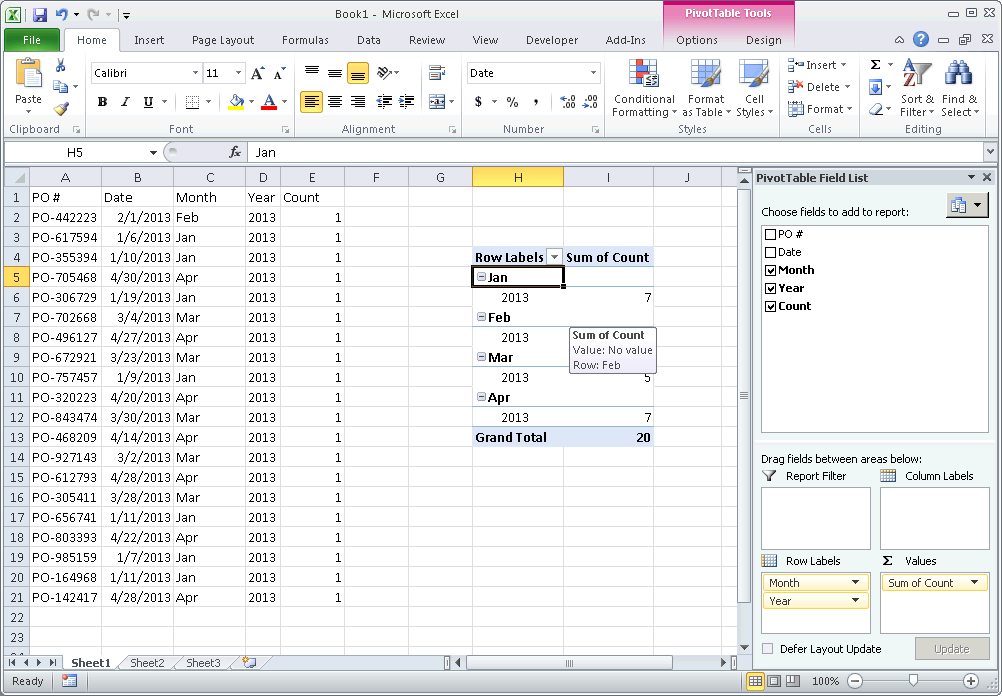# How to count occurrences of each year in Excel?

Apr 20, 2020Normally we can apply the COUNTIF function to count items with criteria in Excel. But, do you know how to count dates by year/month/weekday only? This article will introduce several methods to count the number of occurrences per year/quarter/month/weekday in Excel.

Below we will look at a program in Excel VBA that counts the number of year occurrences.

Situation:Note: Dates are in US Format. Months first, Days second. This type of format depends on your windows regional settings.

1. First, we declare three variables of type Integer. One variable we call yearCount, one variable we call yearAsk, and one variable we call i.

Dim yearCount As Integer, yearAsk As Integer, i As Integer

1. We initialize yearCount with the value 0 and yearAsk with the value of cell C4.

yearCount = 0 yearAsk = Range(“C4”).Value

1. We start a For Next loop.

For i = 1 To 16

1. We now check each date and only if the year of the date equals the entered year in cell C4, we increment yearCount by 1. We use the Year function to get the year of a date.

If year(Cells(i, 1).Value) = yearAsk Then yearCount = yearCount + 1 End If

1. Don't forget to close the loop.

Next i

1. Finally, we display the total year occurrences. We use the & operator to concatenate (join) two strings.

MsgBox yearCount & " occurrences in year " & yearAsk

1. Place your macro in a command button and test it.

Result:Note: because we made yearAsk variable, you can simply count the number of year occurrences of another year by entering another year in cell C4, and clicking on the command button again.

#Tutorial#How To#VBA

How to Write Data to Text File in Excel

How To Loop Through UserForm Controls Dynamically in Excel